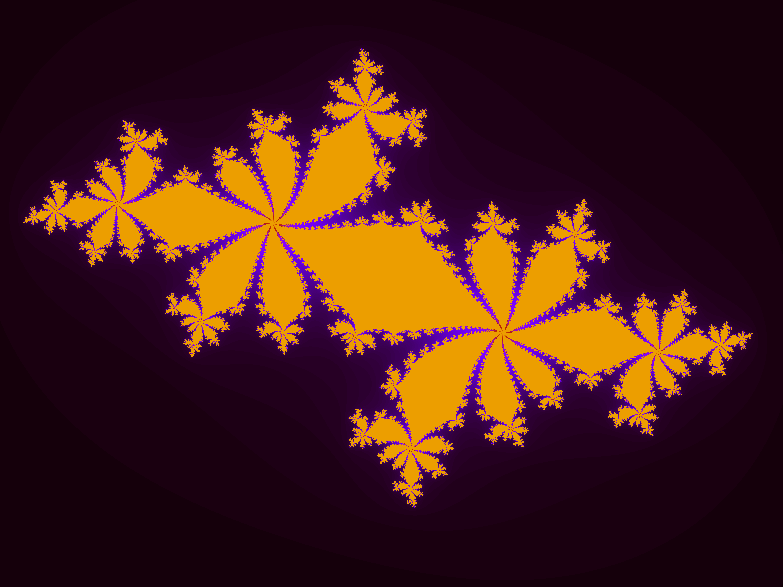# 100 Days of Code Log R1D5

Today I was able to cover a lot of stuff - mainly the exercises and some Gnuplot basics.

I’ve already made a blog post on the first exercise I worked on - Generate Logistic Map in Fortran. It was fun. Also, later I took a look at the solution available at the mooc’s repository. I was really impressed by how the code is organized. Everything looked neat and debuggable. Inspiring code, one could say! Here’s the link to the Julia set code in MOOC Github repository. This is where I came to know that functions in fortran can act upon an array without being specially defined for arrays.

Compared to it, this is my code for Julia set.

 `````` 1 2 3 4 5 6 7 8 9 10 11 12 13 14 15 16 17 18 19 20 21 22 23 24 25 26 27 28 29 30 31 32 33 34 35 36 37 38 39 40 41 42 43 44 45 46 47 48 `````` ``````program juliaset use, intrinsic:: iso_fortran_env, only : dp=>real64, error_unit implicit integer(j-m) complex, parameter :: c = (-0.622772, 0.42193) integer, parameter :: n = 1000 integer :: itr_values(n**2), fn complex(kind=dp) :: z(n**2), zi real(dp) :: x(n), x0, xn x0 = -2._dp xn = 2._dp x=linspace(x0, xn, n) do j=1,n do k=1,n z((j-1)*n + k) = cmplx(x(j), x(k)) end do end do print *, abs(c) do j = 1,n**2 k=1 zi = z(j) do while ((abs(zi) < 2) .and. (k<256)) zi = zi**2 + c k = k+1 end do itr_values(j) = k end do open(newunit=fn, action='write', file='juliaset.dat') do j = 1, n**2 write(fn, *) real(z(j)), aimag(z(j)), itr_values(j) end do close(fn) call execute_command_line('gnuplot -p juliaset.plt') contains function linspace(x0, xn, n) result(x) integer, intent(in) :: n real(dp), intent(in) :: x0, xn real(dp) :: step, x(n) step = (xn-x0)/(n-1) do j=1,n x(j) = x0 + step*(j-1) end do end function linspace end program juliaset ``````

with the following file as juliaset.plt

 ``````1 2 3 4 5 6 7 `````` ``````set nokey set view map set title "Julia Set" set xlabel "Real(z)" set ylabel "Imag(z)" m="juliaset.dat" plot m u 1:2:3 with image ``````

This generated the following plot (cropped here).Apart from this, I had an introduction to the CMake method. As of now, CMakeLists.txt sounds foreign. But, if I am gonna use it further, I may get used to it.